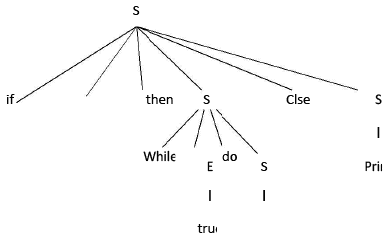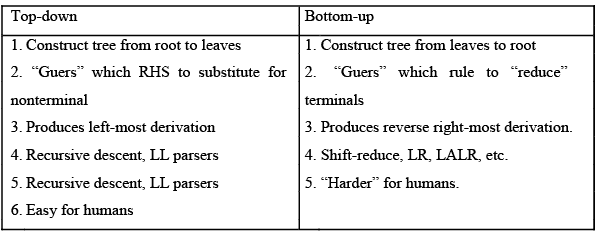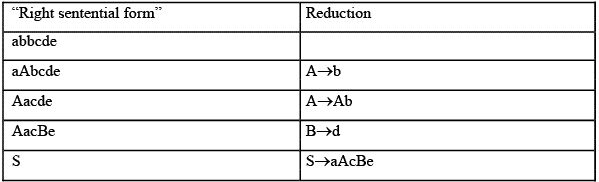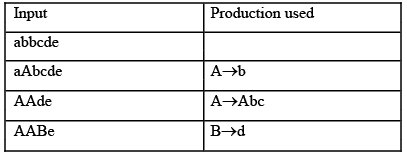Courses

Introduction & Difference Between Top Down & Bottom Up Parsing Notes | Study Compiler Design - Computer Science Engineering (CSE)

Computer Science Engineering (CSE): Introduction & Difference Between Top Down & Bottom Up Parsing Notes | Study Compiler Design - Computer Science Engineering (CSE)

The document Introduction & Difference Between Top Down & Bottom Up Parsing Notes | Study Compiler Design - Computer Science Engineering (CSE) is a part of the Computer Science Engineering (CSE) Course Compiler Design.
All you need of Computer Science Engineering (CSE) at this link: Computer Science Engineering (CSE)

BOTTOM UP PARSING:
Bottom-up parser builds a derivation by working from the input sentence back towards the start symbol S. Right most derivation in reverse order is done in bottom-up parsing.
(The point of parsing is to construct a derivation. A derivation consists of a series of rewrite steps)
S⇒r0⇒r1⇒r2⇒- - - ⇒rn-1⇒rn⇒sentence
Bottom-up

Assuming the production A →b, to reduce ri ri-1 match some RHS b against ri then replace b with its corresponding LHS, A.

In terms of the parse tree, this is working from leaves to root.

Example – 1: S→if E then S else S/while

E do S/ print E→ true/ False/id

Input: if id then while true do print else print.

Parse tree:
Basic idea: Given  input  string  a,  “reduce”  it  to  the  goal  (start)  symbol,  by  looking  for substring that match production RHS.⇒ if E then S else S
lm
⇒ if id then S else S
lm
⇒ if id then while E do S else S
lm
⇒ if id then while true do S else S
lm
⇒ if id then while true do print else S
lm
⇒ if id then while true do print else print
lm
⇒ if E then while true do print else print
rm
⇒ if E then while E do print else print
rm
⇒ if E then while E do S else print
rm
⇒ if E then S else print
rm
⇒ if E then S else S
rm
S
rm

Topdown Vs Bottom-up parsing:→ Bottom-up can parse a larger set of languages than topdown.
→Both work for most (but not all) features of most computer languages.

Example – 2:

Right-most derivation

S → aAcBe          llp  : abbcde/ S              → aAcBe
A→ Ab/b                                                    →aAcde
B→d
→aAbcde
→abbcde

Bottom-up approachSteps correspond to a right-most derivation in reverse.

(must choose RHS wisely)
Example – 3:
S →aABe
A →Abc/b
B →d
1/p:abbcde

Right most derivation:
S →aABe
→aAde Since ( ) B →d
→aAbcde Since ( )A →Abc
→abbcde Since ( ) A →b
Parsing using Bottom-up approach:S parsing is completed as we got a start symbol Hence the 1/p string is acceptable.

Example – 4 E→E+E

E→E*E

E→(E)

E→id 1/p: id1+id2+id3

Right most derivation
E →E+E
→E+E*E
→E+E*id3 →E+id2*

id3 →id1+id2*id3

Parsing using Bottom-up approach:

Go from left to right
id1+id2*id3
E+id2*id3
E+E*id3
E*id3

E*E
E→id
E→id
E→E+E
E→id
E

= start symbol, Hence acceptable.

The document Introduction & Difference Between Top Down & Bottom Up Parsing Notes | Study Compiler Design - Computer Science Engineering (CSE) is a part of the Computer Science Engineering (CSE) Course Compiler Design.
All you need of Computer Science Engineering (CSE) at this link: Computer Science Engineering (CSE)Use Code STAYHOME200 and get INR 200 additional OFF Use Coupon Code

Top Courses for Computer Science Engineering (CSE)Compiler Design

16 videos|44 docs|29 tests

Top Courses for Computer Science Engineering (CSE)Track your progress, build streaks, highlight & save important lessons and more!

,

,

,

,

,

,

,

,

,

,

,

,

,

,

,

,

,

,

,

,

,

;